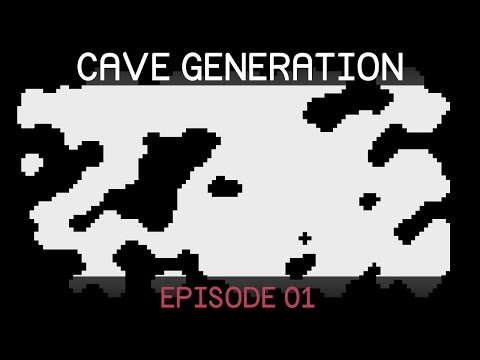# Cellular Automata

#### Difficulty: Advanced

Part 1 of 9: Cellular Automata.## Cellular Automata

Advanced Procedural Cave Generation tutorial

In this lesson a random seed is set using "System.Random". This is a valid approach. It is worth noting that Unity also has a Random class and a Random.seed built in. These can be used as an alternative to System.Random.

### Code snippet

``````using UnityEngine;
using System.Collections;
using System;

public class MapGenerator : MonoBehaviour {

public int width;
public int height;

public string seed;
public bool useRandomSeed;

[Range(0,100)]
public int randomFillPercent;

int[,] map;

void Start() {
GenerateMap();
}

void Update() {
if (Input.GetMouseButtonDown(0)) {
GenerateMap();
}
}

void GenerateMap() {
map = new int[width,height];
RandomFillMap();

for (int i = 0; i < 5; i ++) {
SmoothMap();
}
}

void RandomFillMap() {
if (useRandomSeed) {
seed = Time.time.ToString();
}

System.Random pseudoRandom = new System.Random(seed.GetHashCode());

for (int x = 0; x < width; x ++) {
for (int y = 0; y < height; y ++) {
if (x == 0 || x == width-1 || y == 0 || y == height -1) {
map[x,y] = 1;
}
else {
map[x,y] = (pseudoRandom.Next(0,100) < randomFillPercent)? 1: 0;
}
}
}
}

void SmoothMap() {
for (int x = 0; x < width; x ++) {
for (int y = 0; y < height; y ++) {
int neighbourWallTiles = GetSurroundingWallCount(x,y);

if (neighbourWallTiles > 4)
map[x,y] = 1;
else if (neighbourWallTiles < 4)
map[x,y] = 0;

}
}
}

int GetSurroundingWallCount(int gridX, int gridY) {
int wallCount = 0;
for (int neighbourX = gridX - 1; neighbourX <= gridX + 1; neighbourX ++) {
for (int neighbourY = gridY - 1; neighbourY <= gridY + 1; neighbourY ++) {
if (neighbourX >= 0 && neighbourX < width && neighbourY >= 0 && neighbourY < height) {
if (neighbourX != gridX || neighbourY != gridY) {
wallCount += map[neighbourX,neighbourY];
}
}
else {
wallCount ++;
}
}
}

return wallCount;
}

void OnDrawGizmos() {
if (map != null) {
for (int x = 0; x < width; x ++) {
for (int y = 0; y < height; y ++) {
Gizmos.color = (map[x,y] == 1)?Color.black:Color.white;
Vector3 pos = new Vector3(-width/2 + x + .5f,0, -height/2 + y+.5f);
Gizmos.DrawCube(pos,Vector3.one);
}
}
}
}

}``````

#### Related documentation#### Procedural Cave Generation tutorial

1. Cellular Automata
2. Marching Squares
3. Creating Meshes